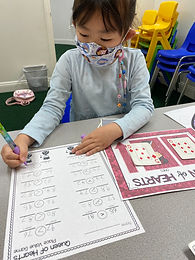Ms. Brianne

Target 1​

Lesson Type:

Continuation

Number Operation

:

Computation

Add within 1,000 (regrouping limited to only one place-value).

1:

Understand why the standard algorithm for adding is based on place-value.

2:

Understand that when adding a multi-digit number, each place-value is being added individually to create the new sum (ones are added to ones, tens are added to tens, etc.)

3:

Understand that when adding a multi-digit number, to solve start in the lowest place-value and go left.

4:

Understand how to regroup based on place-value understandings (i.e., ten ones can be composed into one ten – ten tens can be composed into one hundred).

3rd

Vocabulary:

Activities:

• Students practiced solving addition problems with regrouping.
• Students solved for the sum of 3-digit addition problems, using regrouping when necessary. Then they colored the surrounding heart according to whether the sum was an even or odd number.Home Exploration

Guiding Questions:Absent Students:

Target 2

:

1:

Compare two quantities or numbers up to 20.

2:

Understand the meaning of the symbols > (greater than), < (less than), and = (equal to).

3:

Use place-value understandings to compare numbers.

2nd

Vocabulary:

Compare, Greater Than, Less Than, Equal To

Activities:

• Students reviewed the symbols for greater than (>), less than (<), and equal to (=).
• Students picked two numbers from a deck of cards and used their understandings of place value to create the largest number. Then they compared their numbers, using the appropriate symbol.Home Exploration

Guiding Questions:Target 3

:

1:

Use the given information in a problem to identify the correct answer.

2:

Use the given information to determine which is not the correct answer.

3:

Follow given rules to solve problems.

3rd

Vocabulary:

Logic, Puzzle, Clues, Reasoning

Activities:

• Students used clues from the equations to determine the value of each picture. Then they used those values in other equations to solve for more picture variables.
• Students placed a given number of hearts in a grid so that there are the same number of hearts in each row or column.Home Exploration